# Transformers Sample Assignment

Transformers

1. The turns ratio modified to 20:1, calculate the following values of Vs and Is for the given values of RLin the table below: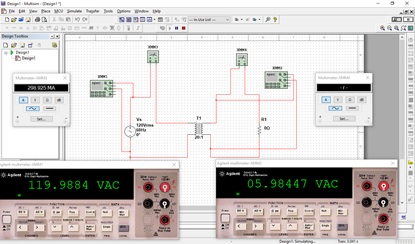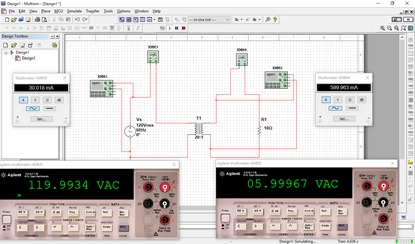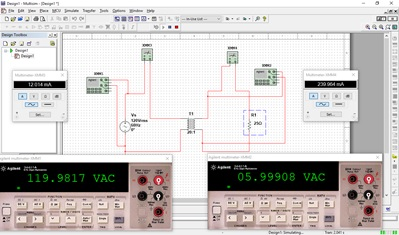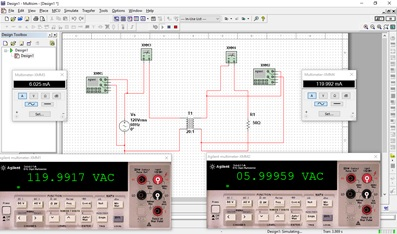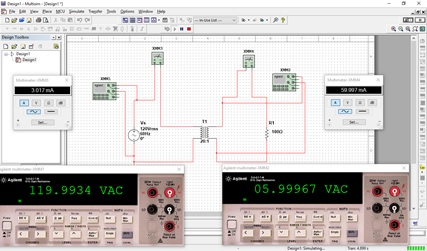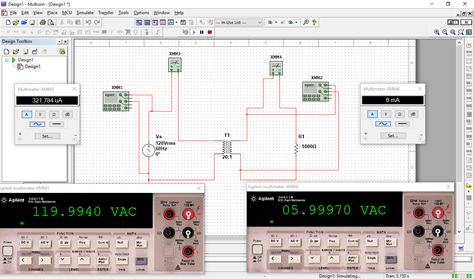RL Calculated Vs Calculated Is Measured Vs Measured Is 0 Ω 120Vrms/20:1 = 6Vrms 6Vrms/0ohms= 0A 05.99Vac -r- 10 Ω 120Vrms/20:1 = 6Vrms 6Vrms/10ohms= 600mA 05.99Vac 599.97mA 25 Ω 120Vrms/20:1 = 6Vrms 6Vrms/25ohms= 240mA 05.99Vac 239.991mA 50 Ω 120Vrms/20:1 = 6Vrms 6Vrms/50ohms= 120mA 05.99Vac 119.995mA 100 Ω 120Vrms/20:1 = 6Vrms 6Vrms/100ohms= 60mA 05.99Vac 59.998mA 1000 Ω 120Vrms/20:1 = 6Vrms 6Vrms/1000ohms= 6mA 05.99Vac 6mA

2. Construct the transformer circuit in MultiSIM and complete the table in part 2 with the measured values of Vs and Is for each value of RLUse the Agilent Digital Multi-meter to take the measurements and capture a screen shot of each measurement.

3. Discuss the following: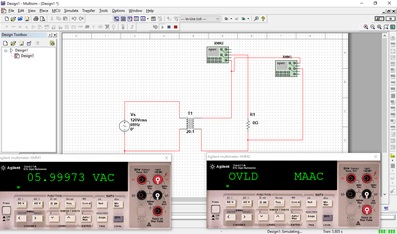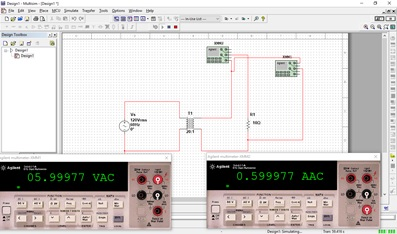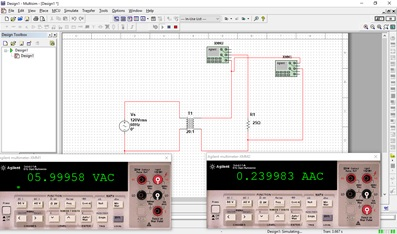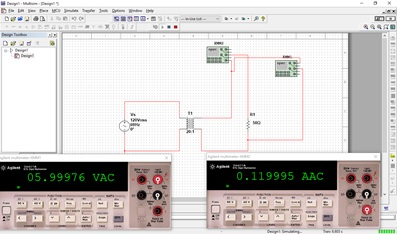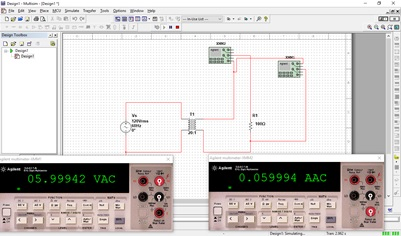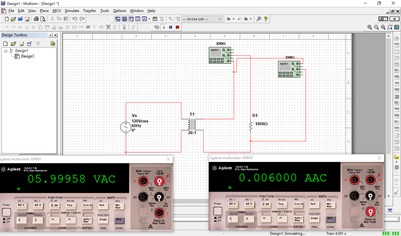• Describe the relationship of RLon the secondary voltage.

The secondary voltage equals RL times Is.

1. Describe the relationship of RLon the secondary current.

The secondary current equals secondary voltage divided by RL

2. How do the calculated values compare to the measured values?

Yes, although I can get a 0A when resistor equals 0 but when measuring I get –r- or overload reading.  Np/Ns = Vp/Vs.

3. How would you go about calculating the transformer power efficiency?

Efficiency = (Vs*Is)/(Vp*Ip)

4. How would you go about measuring the transformer power efficiency?

Put 2 wattmeter on both ends of the transformer (on the primary and on the secondary). Divide the output of power of primary by the input power of the secondary.

5. Determine the transformer power efficiency for the circuit in MultiSIM. Include a calculation and a measured value.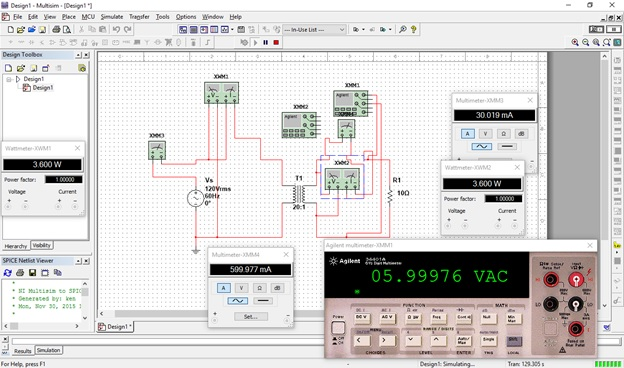Efficiency = (Vs*Is)/(Vp*Ip)= (5.99*0.599)/(120*0.030) = (3.58/3.60)

= 99.44%

Include all calculations, screenshots of measurements, the table results of part 2 and the answers to part 4 in a word processing document and submit as EE115W4LabYourGID.docx, or an equivalent word processing file extension.